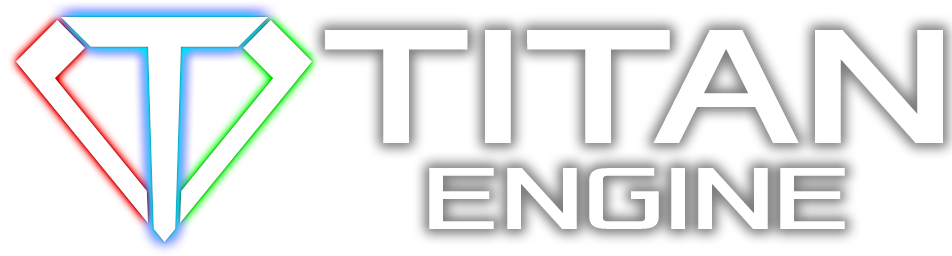About Store Forum Documentation ContactVertex skinning Author Message
JayJayMember

 Post: #1 Vertex skinning Model B is a copy of model A except that some vertices were slightly moved to a nother position (but no face, edge or vertex was deleted or added). A skeleton was added to model A using the model editor. Is it possible to map the vertex skinning of model A to model B? How is the vertex-skinning-data stored? Can I access the vertex-skinning-data in C++? Thanks in advance! (This post was last modified: 08-18-2011 02:55 PM by JayJay.)
08-18-2011 02:49 PM
EsenthelJayJayPost: #3 RE: Vertex skinning Thank you Esenthel. If someone wants to do something like this: The easiest way in C++ to copy all data from model A to model B except vtx-positions (or what ever): 1) create a copy C of mesh A 2) replace the vtx-positions (or what ever you want) of C with the ones of B PHP Code: `for (int j = 0; j < to->parts.elms() && j < from->parts.elms(); j++){    for (int k = 0; k < to->parts[j].base.vtxs() && k < from->parts[j].base.vtxs(); k++)    {        // copy the position        to->parts[j].base.vtx.pos(k) = from->parts[j].base.vtx.pos(k);    }      // recalculate vertex normals    to->parts[j].base.setNormals();} ` 3) replace B with C It's that easy. Note that this just works if model B is a copy of model A except that some vertices were slightly moved to a nother position. (This post was last modified: 08-19-2011 01:17 PM by JayJay.)